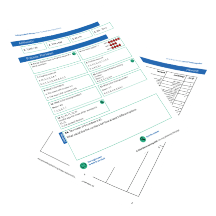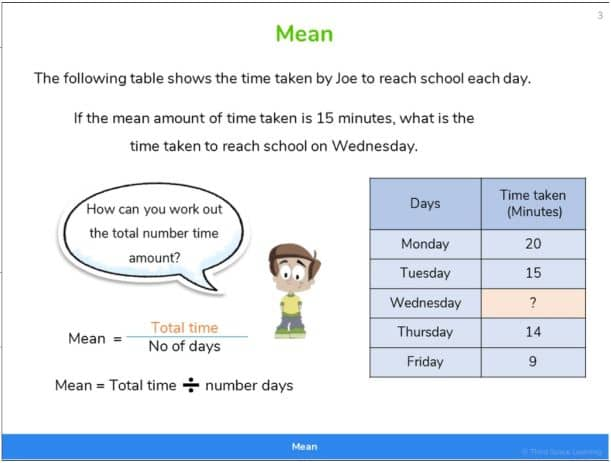Teaching support from the UK’s largest provider of in-school maths tuitionone to one lessonsschools supported

Online one to one tuition designed to boost confidence, engagement and verbal fluency

Hundreds of FREE online maths resources!

Daily activities, ready-to-go lesson slides, SATs revision packs, video CPD and more!Explore all resources
Key Stage 2 (Other Topics)

Online one to one tuition designed to boost confidence, engagement and verbal fluency

# What Are Mean Median Mode Range? Definition, Examples & Practice Question For Primary School

Find out what mean, median, mode and range are, when they are taught in school, and how you can help children to understand them as part of their maths learning at home.

Before the 2014 curriculum reforms, pupils were expected to learn about all 4 different types of averages and solve problems based on their application. But now the national curriculum only has ‘mean’ as a type of average that pupils need to be explicitly taught in Year 6.

Despite this, some schools continue to teach all four different averages after Year 6 have completed their end-of-year assessments.

## What are mean, median, and mode?

Mean mode and medium are different types of averages from a data set.

### What is mean?

The mean is the average of a data set. It can be calculated by adding up all of the numbers in the data set and then dividing by the total number of values in the set.

It is also known as ‘arithmetic mean’, but NOT a ‘geometric mean’.

### What is median?

The median is the middle value (or midpoint) when a data set is ordered from least to greatest.

So list the numbers in your dataset from the lowest value to the highest value. The median is the number that is in the middle of the list of numbers.

### What is mode?

The mode is the value that appears the most number of times in a set of data.

So identify how many number of numbers appear in your dataset, and which particular number appears most often. You can use a frequency table to work this out.

### What is range?

The range is the difference between the largest value and the smallest value.

To calculate the range, take away the smallest value in your set of values from the largest. The number you are left with is your range.

## Mean median mode and range in the national curriculum

It is useful for pupils to be aware of mean, median, mode and range despite it not being an explicit requirement. Introducing the concepts at this stage proves useful in later years. For example, when dealing with data that contains outliers, finding the mean may not always be the best option.FREE Independent Recap Mean Worksheet

This time-saving worksheet is for Year 6 pupils and includes 4 arithmetic questions, 9 consolidation questions, and 1 challenge question.Download Free Now!

### Mean median mode examples

To find an average, you must first have a data set. The following data set is from a game a group of pupils were playing in the classroom.

To calculate the mean, median, mode and range, we need to manipulate this data using the information about how to find each particular average above.

### Finding the mean

Taking the above data as an example, to find the mean you would need to add 7, 12, 15, 7 and 4 together to get 45 and then divide this by the number of values, in this case, 5. The average value is 9. With bigger numbers, pupils can use partitioning to help them with the calculations.

Due to the amount of calculating involved in finding the mean, it is referred to as the ‘meanest’ of the averages. This is one way you can remind pupils how to find the mean of a number of data points!

### Finding the mode

With our set of numbers, the mode would be 7 as this appears twice in our data in the table above. Mode shares some similar orthography with the word ‘most’ which can be a useful way to get pupils to remember how to find the mode.

### Finding the median

The median value is found by finding the middle value. Remember that the data needs to be placed into value order. That means we take the data values from the table and write them out in ascending order. Once that is done, our data looks like this:

4, 7, 7, 12, 15

The middle value, or median, is 7 as there are an equal number of other values on either side of the second 7. When your data set contains an even number of values rather than an odd number, take the pair of numbers that would make the median, add them together and then divide them by 2.

### Finding the range

The range is the difference between the highest and lowest values. With our data set that would be 11, as the highest number is 15 (Yahyah) and the lowest number is 4 (Christine). To find the difference, you need to subtract the highest value from the lowest value.

### When do children learn about mean median mode?

There is no longer a requirement for median, mode and range to be taught at the primary phase of school.

Although mean is compulsory in Year 6 and comes under the statistics section of the national curriculum for mathematics, where it states that ‘pupils should be taught to calculate and interpret the mean as an average.’ Median and mode are not part of the national curriculum in the UK.

Instead, the non-statutory guidelines state that pupils should know when it is appropriate to find the mean of a data set.A Year 6 lesson slide teaching problem solving and findingthe mean on Third Space Learning’s online intervention.

### How does mean median mode relate to other areas of maths?

While there is no requirement for it to be linked to other areas of mathematics, some teachers may choose to give tasks that find the averages of particular data sets. This could include, for example, finding the mean of certain measurable characteristics of the class. For example, hand span, height or shoe size. This data could be obtained during a statistics lesson or a lesson on measurement.

The Office for National Statistics uses the mean to find the mean age of the population. Any role that involves looking at statistical data will likely use all the above measures of central tendency to help draw conclusions from the data.

People may also use the mode or modal value to estimate how long it takes them to do tasks they complete frequently.

For example, if you timed yourself vacuuming where you lived and collected these times in minutes (10, 10, 8, 13, 10), you could say that it takes you around 10 minutes to hoover where you live as 10 is the value with the highest frequency in the data set.

### Mean median mode worked example

Let’s look at a step-by-step mean median mode example question!

Use the data above to find the mean, median, mode and range of the data.

To find the mean, first, add all the data sets.

To solve this we need to add 8, 12, 7, 7, 5 and 15. This is 54.

Next, we divide by the number of data sets. As this data is for 6 people, the number of data sets is 6. This means we need to divide 54 by 6 or know that 6 x ? = 54. This is 9. The mean is 9.

To find the median, we need to re-write the data in order from least to greatest.

5, 7, 7, 8, 12, 15

We then need to find the middle value. As the data set has an even number we need to find the two middle numbers, add them and divide by 2.

The middle numbers (or median numbers) are 7 and 8 as there are two numbers on either side of them. Adding them up together gives us 15. 15 divided by 2 = 7.5.

To find the mode, we are looking for the data that appears most often. 7 is the only whole number that appears more than once, so the mode is 7.

To find the range, we subtract the lowest value from the data set from the highest. The lowest value is 5 and the highest is 15. 15 – 5 = 10. The range is 10.

### Mean median mode practice question

Use the data above, and the examples from further up the page, to find the mean, median, mode and range of the data.

1. Mean is 7
2. Median is 7
3. Mode is 4
4. Range is 7 (11 – 4 = 7)
What’s the difference between mean median mode and range?

Mean median mode and range all calculate the averages of data sets. However, they each use different methods.
Mean is the average of all of the numbers.
Median is the middle number, when in order.
Mode is the most common number.
Range is the largest number minus the smallest number.

How do you find the mean?

Add all of the numbers together and then divide that by how many numbers there are.

What is a median example?

The median is the number in the middle of a data set. For example, in the data set: 7, 6, 9, 2, 1, the median would be 6 as the numbers would be placed in order (1, 2, 6, 7, 9), and 6 is the number in the middle.

Wondering how to explain other key maths vocabulary to your children? Check out our Primary Maths Dictionary, or try these other blogs:

You can find plenty of statistics worksheets for primary school pupils on the Third Space Learning Maths Hub

Online 1-to-1 maths lessons trusted by schools and teachers
Every week Third Space Learning’s maths specialist tutors support thousands of primary school children with weekly online 1-to-1 lessons and maths interventions. Since 2013 we’ve helped over 150,000 children become more confident, able mathematicians. Learn more or request a personalised quote to speak to us about your needs and how we can help.

Primary school tuition targeted to the needs of each child and closely following the National Curriculum.

Related Articles

x#### FREE Independent Recap Mean Worksheet

This time-saving worksheet is for Year 6 pupils and includes 4 arithmetic questions, 9 consolidation questions, and 1 challenge question.

#### FREE Independent Recap Mean WorksheetThis time-saving worksheet is for Year 6 pupils and includes 4 arithmetic questions, 9 consolidation questions, and 1 challenge question.